# Analog-to-Digital Converters Information

Analog-to-digital converters (ADC) sample an analog signal and convert it to a series of digital values. Most data packages in real-world applications are transmitted using analog signals. In order to be read, manipulated, and analyzed by microprocessors, these signals must be converted to digital information. Analog-to-digital converters exist to accomplish this process.

## Sampling

A primary stumbling block to analog-to-digital conversion is the difference between analog and digital signals. Analog signals tend to take the form of a continuous wave, while digital ones are purely numerical and, when graphed, take the form of a square wave. Therefore, digital signals may be mapped to analog ones using sampling, the process of measuring the signal at predetermined times.

There are two important parameters related to sampling:

• The sampling rate, fs, determines the number of samples taken per second.
• Sampling precision, N, determines the gradations for the sampling process. (In other words, sampling precision dictates the y-axis divisions of a graphed signal.)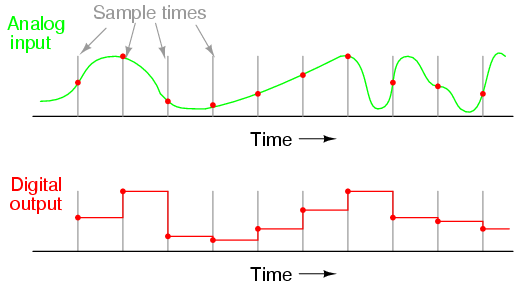Sample rate error. Image credit: Tony R. Kuphaldt

The image above shows a sample rate which is too slow for the changes in the analog signal. In the latter half of the signal mapping, especially, the digital signal fails to accurately reproduce the analog one because the analog signal experiences rapid amplitude changes between samples. By doubling the sample rate, the digital signal would begin to look much more like the original analog one.

Sampling must also be accurately timed to faithfully reproduce analog signals. For example, the image below shows three different sample rates: from top to bottom, f, 2f, and 4f/3. The top example, which shows sampling at once per cycle, is obviously erroneous, and the bottom example has created an inaccurate alias wave because f is larger than the signal's Nyquist frequency (1/2 of the sampling rate). Only the middle example comes close to replicating the signal, but its accuracy is insufficient. It is only by using more frequent sampling—such as that which approaches 5f or greater—that a digital signal can replicate an analog one.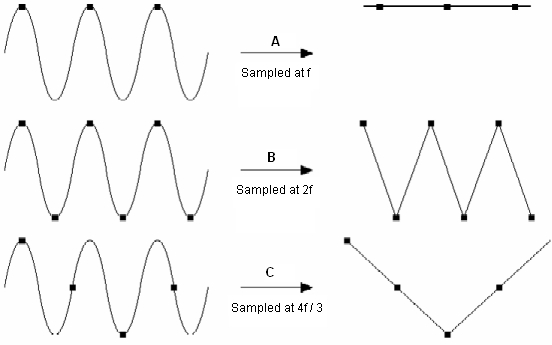Sample rate effects. Image credit: National Instruments

Generally, sampling as quickly as possible with a reasonably fast converter produces the best signal reproduction.

## Resolution and Quality

Analog-to-digital converter precision is determined by the number of bits the device possesses. The number of bits, n, is directly related to the number of sampling gradations, N, so that N = 2n. (Gradations can be thought of as the scale of measured intervals with which to express values contained in the analog signal.)

For example, a 12-bit converter is capable of 4096 gradations. If the analog signal is within the range of 0-5 V (a typical control signal), this range would be broken up into 4096 intervals in order to express analog values, with 0 V being 0 and 5 V being 4095. If the analog signal measures 2.4414 V at a given sample time, the digital equivalent would be 2000. The entire analog signal can be mapped proportionally using these gradations.

Device precision can be defined as 1/N, with smaller values indicating greater precision. For example, a 4-bit converter has a precision 1/16 that of the analog signal, while a 10-bit one is 1/1024 as precise.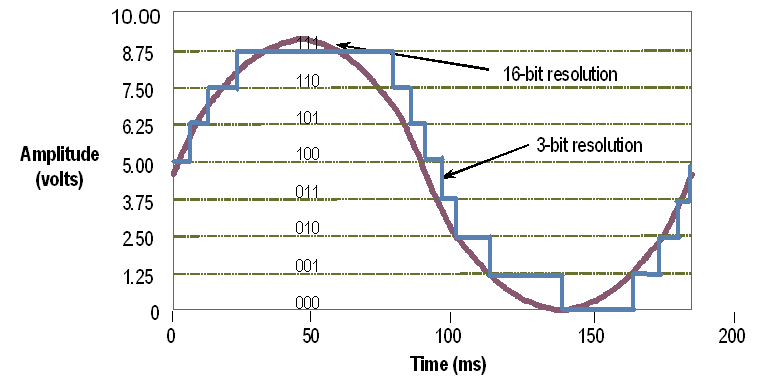Comparison of a sine wave translated using 16-bit (purple) and 3-bit (blue) resolutions. The 16-bit device is capable of 65,528 more gradations than the 3-bit one, resulting in a much more accurate signal. Image credit: National Instruments

## Converter Design and Specifications

The basic building block of most analog-to-digital converters is the comparator, a device similar in construction (but not identical) to an operational amplifier. Comparators accept differential analog inputs and output a proportional digital signal, so that a comparator alone could operate as a 1-bit ADC.

Some examples of common ADC types are listed in the table below.

 Type of ADC Description Advantages Disadvantages Image Flash Uses comparator banks to sample input in parallel; typically 8-bit or less due to cumbersome construction. Extremely fast Large form factor, high capacitance, prone to out- of-sequence code glitches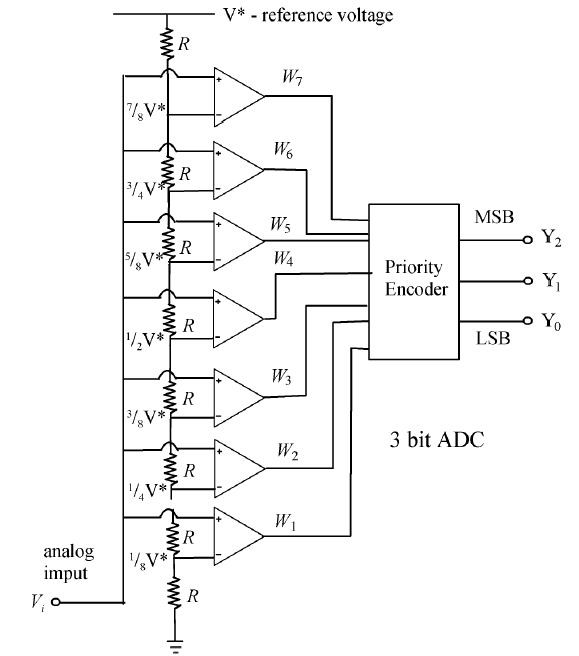Successive-approximation Uses a comparator to successively narrow signal range to replicate analog signal. Power and space efficient Slower than flash types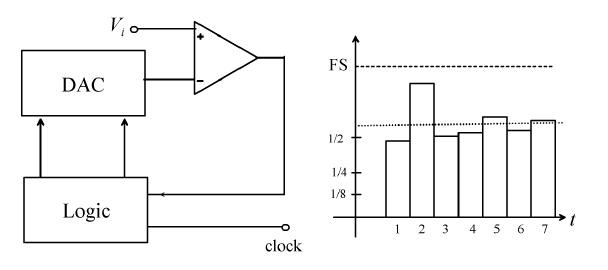Ramp-compare Uses a timed digital signal which is matched to analog signal; timer’s value records analog signal values. Can compare multiple signals simultaneously without second device Timing is temperature- sensitive and may lead to errors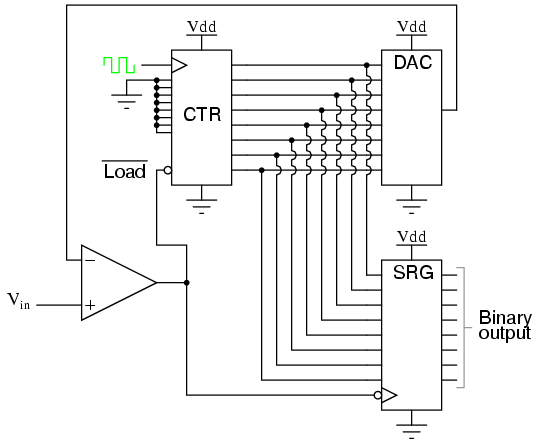Wilkinson Uses a charging capacitor which discharges when input voltage reaches a certain point. A gate pulse is then formed and timed to determine analog amplitude. Superior channel profile and differential linearity Slow speeds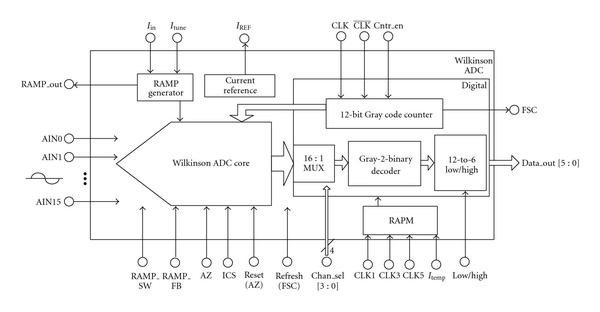Integrating Uses an integrator and a reference voltage to compare clock speeds and determine analog values. Highly accurate Improved resolution requires slower operationTable image credits: Elettronica | All About Circuits | Hindawi Publishing | National Instruments

### Inputs

ADCs are often manufactured to accept several inputs, with some larger data acquisition or I/O instruments accepting 50 or more. Some devices include differential input channels, in which the difference between two analog inputs is measured. Differential inputs are useful for comparing minute differences between two input signals, such as low voltages.

### Form Factor

Analog-to-digital converters take various forms, including PCB modules (shown at bottom right), rack-mount, DIN rail, and panel-mount. Form factor is typically important due to measurements and space requirements; for example, rack-mount devices are typically standardized at 19" widths, while DIN rail converters meet the guidelines for device sizes as laid out in the Deutsches Institut für Normung (DIN) standards.

## Standards

Several aspects of analog-to-digital converters, including terminology, design, use, and testing, may be governed by published standards. Examples of major standards include: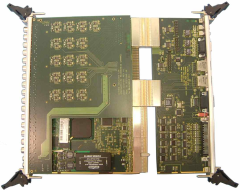## References

Analog Dialogue - The Data Conversion Handbook

#### Image credits: